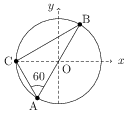# Electric Field and Potential

## Electric Field of a Point Charge

Consider a charge $q$ fixed at the point O. Let P be a point at a distance $r$ from O. The electric field of charge $q$ at the point P is given by \begin{align} \vec{E}=\frac{1}{4\pi\epsilon_0}\frac{q}{r^2} \hat{r} \end{align} where $\epsilon_0$ is permittivity of free space ($1/4\pi\epsilon_0=9\times{10}^{9}\;$ m/F) and $\hat{r}$ is the unit vector in the direction from O to P.

## Principle of Superposition

The electric field of a system of fixed point charges is equal to the vector sum of the electric fields that would be created by each charge separately: \begin{align} \vec{E}=\sum \vec{E}_i=\frac{1}{4\pi\epsilon_0}\frac{q_i}{r_i^2}\hat{r}_i \end{align}

## The field on the axis of a thin uniformly charged ring

A charge $q>0$ is uniformly distributed over a thin ring of radius $a$. The electric field $\vec{E}$ on the axis of the ring as a function of distance $x$ from its centre is given by, \begin{align} |\vec{E}|=\frac{q}{4\pi\epsilon_0} \frac{x}{(a^2+x^2)^{3/2}}. \end{align}

## The field of a uniformly charged straight filament

A thin straight filament of length $2l$ is uniformly charged by a charge $q$. The field at a point separated by a distance $x$ from the midpoint of the filament and located symmetrically with respect to its ends is given by \begin{align} |\vec{E}|=\frac{q}{4\pi\epsilon_0 x} \frac{1}{\sqrt{l^2+x^2}}. \end{align}

## Problems from IIT JEE

Problem (IIT JEE 2008):Consider a system of three charges $\frac{q}{3}$, $\frac{q}{3}$ and $-\frac{2q}{3}$ placed at points A, B and C, respectively, as shown in the figure. Take O to be the centre of the circle of radius $R$ and angle $\text{CAB}={60}\;\mathrm{degree}$.

1. The electric field at point O is $\frac{q}{8\pi\epsilon_0 R^2}$ directed along the negative $x$-axis.
2. The potential energy of the system is zero.
3. The magnitude of the force between the charge C and B is $\frac{q^2}{54\pi\epsilon_0 R^2}$.
4. The potential at point O is $\frac{q}{12\pi\epsilon_0 R}$.

Solution: The charges at A, B, and C are $q_A=q/3$, $q_B=q/3$, and $q_C=-2q/3$. The electric field at O due to $q_A$ and $q_B$ is equal in magnitude but opposite in direction. Thus, resultant electric field at O is by the charge $q_C$ and it is given by \begin{alignat}{2} \vec{E}_O=-\frac{q}{6\pi\epsilon_0 R^2}\;\hat\imath. \nonumber \end{alignat} The triangle ABC is right-angled with $\angle A={60}\;\mathrm{deg}$, $\angle C={90}\;\mathrm{deg}$, and $r_\text{AB}=2R$. Thus, $r_\text{AC}=R$ and $r_\text{BC}=\sqrt{3}R$. The potential energy for given charge distribution is \begin{alignat}{2} U&=\frac{1}{4\pi\epsilon_0}\left[ \frac{q_A q_B}{r_\text{AB}}+\frac{q_A q_C}{r_\text{AC}}+\frac{q_B q_C}{r_\text{BC}}\right] \nonumber\\ &=\frac{1}{4\pi\epsilon_0} \left[\frac{q^2}{18R}-\frac{2q^2}{9R}-\frac{2q^2}{9\sqrt{3}R}\right]\neq 0. \nonumber \end{alignat} The magnitude of force between $q_C$ and $q_B$ is $F_\text{BC}=\frac{1}{4\pi\epsilon_0}\frac{q_B q_C}{r_\text{BC}^2}=\frac{q^2}{54\pi\epsilon_0 R^2}$. The potential at O is $V=\frac{1}{4\pi\epsilon_0}(q_A/R+q_B/R+q_C/R)=0$.